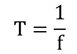# AC (Alternating Current) Circuits

The voltage and current periodically reverses in direction in a regular manner in the alternating system. The amount of voltage changes with time and the polarity changes regularly. The sinusoidal waveform is the commonly used signal waveform in electrical and electronic engineering, which is shown in the figure below. The horizontal line across the centre is the zero axis. The AC passes through a definite sequence and the complete sequence is called a cycle and the waveform is called as a sine wave. The positive and negative parts are equal when the average is zero.

FIGURE

The number of cycles occurring in one second is called as the frequency (f) and its unit is Hertz (Hz). The time taken to complete one cycle is known as the periodic time (T), which is calculated using the formula below.### Types of Periodic Waveforms

Some waveforms are complicated and are produced by audio amplifiers that deals with analog voice signals. In case of symmetrical triangular waveforms, a quarter cycle represents all four quarter cycles of the waveform. The square waveform is used in digital and radar systems. These three general types of AC voltage waveforms are shown in the figure below.

FIGURE